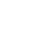Cohort analysis is super important if you want to know if your service is in fact a leaky bucket despite nice growth of absolute numbers. There’s a good write up on that subject “Cohorts, Retention, Churn, ARPU” by Matt Johnson.

So how to do it using R and how to visualize it. Inspired by examples described in “Retention, Cohorts, and Visualizations” I came up with the following solution.

First, get the data in a suitable format, like this:

```cohort  signed_up  active_m0  active_m1  active_m2
2011-10 12345      10432      8765       6754
2011-11 12345      10432      8765       6754
2011-12 12345      10432      8765       6754```

Cohort here is in “YYYY-MM” format, signed_up is the number of users who have created accounts in the given month, active_m0 – number of users who have been active in the same month as they registered, active_m1 – number of users who have been active in the following month, and so forth. For newest cohorts you’ll be getting zeroes in some of active_mN columns, since there’s no data on them yet. This is taken into account in processing scripts.

```require(plyr)

con = gzcon(url('http://www.systematicportfolio.com/sit.gz', 'rb'))
source(con)
close(con)

cohorts
# Let's convert absolute values to percentages (% of the registered users remaining active)
cohort_p as.numeric(df\$active_m0/df\$signed_up), as.numeric(df\$active_m1/df\$signed_up), as.numeric(df\$active_m2/df\$signed_up),
as.numeric(df\$active_m3/df\$signed_up), as.numeric(df\$active_m4/df\$signed_up), as.numeric(df\$active_m5/df\$signed_up),
as.numeric(df\$active_m6/df\$signed_up), as.numeric(df\$active_m7/df\$signed_up), as.numeric(df\$active_m8/df\$signed_up) ))

# Create a matrix
temp = as.matrix(cohort_p[,3:(length(cohort_p[1,])-1)])
colnames(temp) = paste('Month', 0:(length(temp[1,])-1), sep=' ')
rownames(temp) = as.vector(cohort_p\$V1)

# Drop 0 values and format data
temp[] = plota.format(100 * as.numeric(temp), 0, '', '%')
temp[temp == " 0%"] # Plot cohort analysis table
plot.table(temp, smain='Cohort(users)', highlight = TRUE, colorbar = TRUE)
```

This code produces nice visualizations of the cohort analysis as a table:I used articles “Visualizing Tables with plot.table” and “Response to Flowingdata Challenge: Graphing obesity trends” as an inspiration for this R code.

If you want to get nice colours as in the example above, you’ll need to adjust rainbow interval for plot.table. I managed to do it by editing functions code directly from R environment:

`plot.table.helper.color <- edit(plot.table.helper.color)`
```function
(
temp # matrix to plot
){
# convert temp to numerical matrix
temp = matrix(as.double(gsub('[%,\$]', '', temp)), nrow(temp), ncol(temp))

highlight = as.vector(temp)
cols = rep(NA, len(highlight))
ncols = len(highlight[!is.na(highlight)])
cols[1:ncols] = rainbow(ncols, start = 0, end = 0.3)

o = sort.list(highlight, na.last = TRUE, decreasing = FALSE)
o1 = sort.list(o, na.last = TRUE, decreasing = FALSE)
highlight = matrix(cols[o1], nrow = nrow(temp))
highlight[is.na(temp)] = NA
return(highlight)
}
```

Adjust interval in line 11 to 0.5, 0.6 to get shades of blue.
plot.table.helper.colorbar <- edit(plot.table.helper.colorbar)

```function
(
plot.matrix # matrix to plot
)
{
nr = nrow(plot.matrix) + 1
nc = ncol(plot.matrix) + 1

c = nc
r1 = 1
r2 = nr

rect((2*(c - 1) + .5), -(r1 - .5), (2*c + .5), -(r2 + .5), col='white', border='white')
rect((2*(c - 1) + .5), -(r1 - .5), (2*(c - 1) + .5), -(r2 + .5), col='black', border='black')

y1= c( -(r2) : -(r1) )

graphics::image(x = c( (2*(c - 1) + 1.5) : (2*c + 0.5) ),
y = y1,
z = t(matrix( y1 , ncol = 1)),
col = t(matrix( rainbow(len( y1 ), start = 0.5, end = 0.6) , ncol = 1)),
}
```

Adjust interval in line 21 to 0.5, 0.6 to get shades of blue.

Now if you want to draw the cycle-like graph:

```# make matrix shorter for the graph (limit to 0-6 months)
temp = as.matrix(cohort_p[,3:(length(cohort_p[1,])-1)])
temp temp[temp == "0"]
library(RColorBrewer)
colnames(temp) = paste('Month', 0:(length(temp[1,])-1), 'retention', sep=' ')
palplot(temp[,1],pch=19,xaxt="n",col=pal,type="o",ylim=c(0,as.numeric(max(temp[,-2],na.rm=T))),xlab="Cohort by Month",ylab="Retention",main="Retention by Cohort")

for(i in 2:length(colnames(temp))) {
points(temp[,i],pch=19,xaxt="n",col=pal[i])
lines(temp[,i],pch=19,xaxt="n",col=pal[i])
}

axis(1,at=1:length(cohort_p\$cohort),labels=as.vector(cohort_p\$cohort),cex.axis=0.75)
legend("bottomleft",legend=colnames(temp),col=pal,lty=1,pch=19,bty="n")
abline(h=(seq(0,1,0.1)), col="lightgray", lty="dotted")
```

This code produces nice visualizations of the cohort analysis as multicolour cycle graph:## 4 thoughts on “Measuring user retention using cohort analysis with R”

1.David Linder says:

This is great stuff. I’ve been asked to do produce subscription-based cohort models, i.e. How many people signed up? vs How many people subscribed in the following days? I’ve been doing most of my work in Excel, but I’m finding it hard to automate. Your code looks like something I’ll be able to learn a lot from. Aside from R, do you have any recommendations for how you could build something in Microsoft office after pulling data from a SQL query? Thanks for sharing!

2.Faisal Al-Khalidi says:

Great piece, Ivan! Thanks for this 🙂

3.max says: